# Paasche Price Index

Measures the change in the price and quantity of a basket of goods and services relative to a base year price and quantity

## What is the Paasche Price Index?

The Paasche Price Index is a consumer price index used to measure the change in the price and quantity of a basket of goods and services relative to a base year price and observation year quantity. Developed by German economist Hermann Paasche, the Paasche Price Index is commonly referred to as the “current weighted index.”### Understanding the Paasche Price Index

The Paasche Price Index is a price index used to measure the general price level and cost of living in the economy and to calculate inflation. The index commonly uses a base year of 100, with periods of higher price levels shown by an index greater than 100 and periods of lower price levels with indexes lower than 100.

The Paasche Price Index is commonly confused with the Laspeyres Price Index. The main differentiator between the Paasche Index and the Laspeyres Price Index is that the former uses current-period quantity weightings while the latter uses base-period quantity weightings.

### Formula for the Paasche Price Index

The formula for the Paasche Price Index is as follows: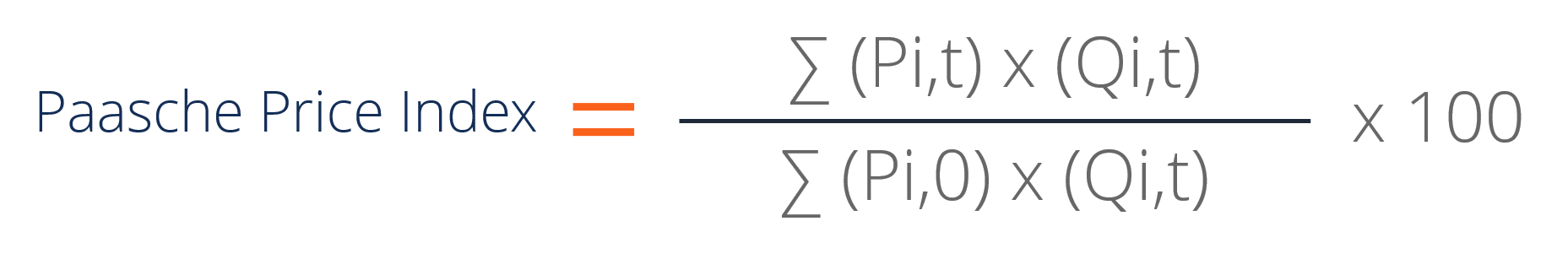Where:

• Pi,0 is the price of the individual item at the base period and Pi,t is the price of the individual item at the observation period.
• Qi,t is the quantity of the individual item at the observation period.

Although the mathematical equation for the Paasche Price Index seems confusing, the numerator is simply the total expenditure for all items at the observation period using current observation period quantities and prices while the denominator is the total expenditure for all items at the base period using current observation period quantities and base period prices. Therefore, the Paasche Price Index can be more easily understood when rewritten as follows: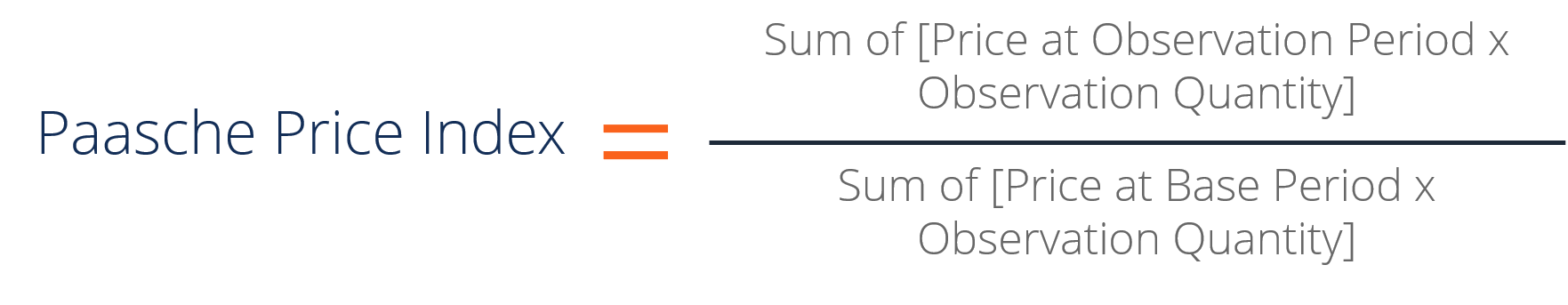### Example of the Paasche Price Index

The following information regarding the change in the prices and quantities of each individual good in a hypothetical economy is provided. Determine the Paasche Price Index for Year 0, Year 1, and Year 2, using Year 0 as the base year.

 Item Year 0 Year 1 Year 2 Good A \$5 \$6 \$7 Good B \$10 \$11 \$13 Good C \$20 \$22 \$24

 Item Year 0 Year 1 Year 2 Good A 50 55 57 Good B 60 65 67 Good C 70 75 77

Using the formula for the Paasche Price Index: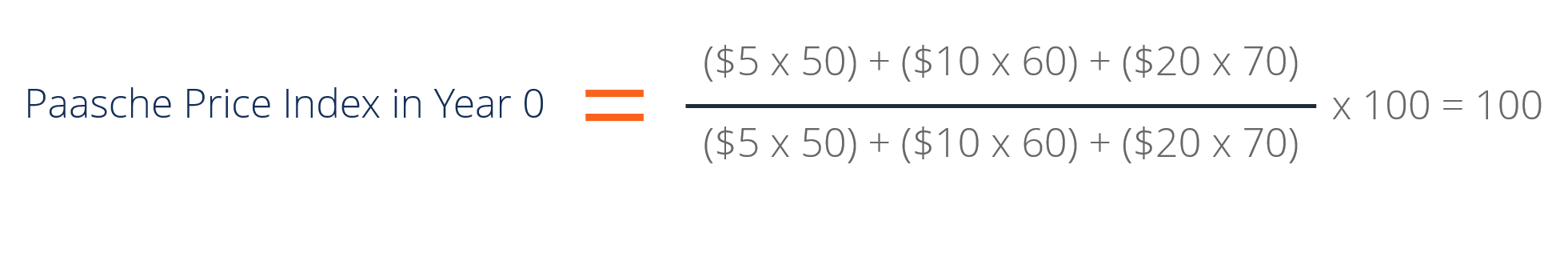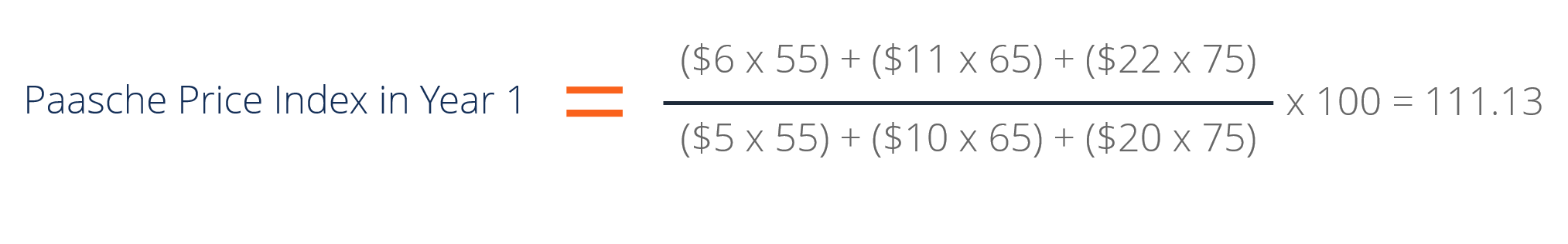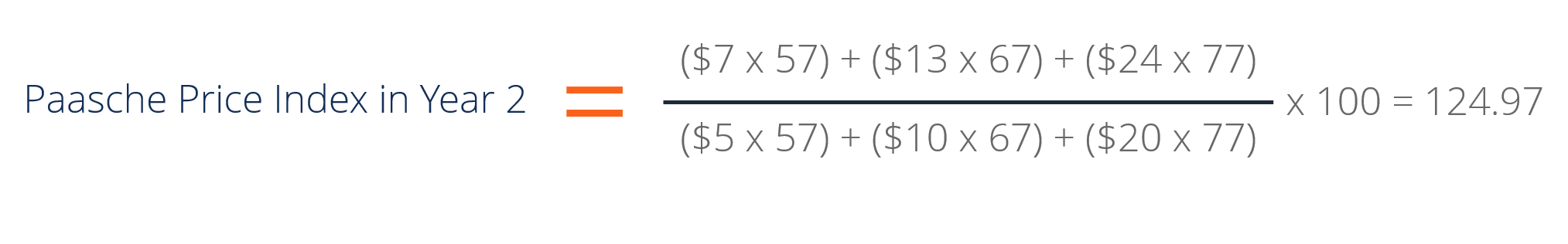Therefore, the price index using the Paasche Price Index is as follows for each year:

• Year 0 (Base Year) = 100
• Year 1 = 111.13
• Year 2 = 124.97

Note that in the Paasche Price Index, the prices are the only items that change. We are comparing the current year (observation year) prices to the base year prices at the same quantities.

### Advantages and Disadvantages of the Paasche Price Index

The advantages of the Paasche Price Index includes:

• Takes into consideration consumption patterns by using current quantities (current weightings)
• Is not upward-biased in terms of price increases (compared to the Laspeyres Price Index)

The disadvantages of the Paasche Price Index includes:

• Data on current weightings (i.e., quantities for each item) can be difficult to obtain
• Costlier than using a Laspeyres Price Index
• Tends to understate the changes in price since the index already reflects changes in consumption patterns when consumer respond to price changes

### Key Takeaways

The Paasche Price Index is a price index used to measure the change in the prices and quantities of a basket of goods and services relative to a specified base period price. The numerator of the index is the total expenditures of all items at the observation period using the observation period price and quantities while the denominator is the total expenditures of all items using base period prices and observation period quantities.

The Paasche Price Index corrects for the upward price bias of the Laspeyres Price Index and also takes into consideration consumption patterns. However, it relies on current data (which may not be readily available) and tends to understate the changes in price.

### Additional Resources

CFI is the official provider of the Financial Modeling and Valuation Analyst (FMVA)™ certification program, designed to transform anyone into a world-class financial analyst.

To keep learning and developing your knowledge of financial analysis, we highly recommend the additional resources below:

• Aggregate Supply and Demand
• Consumer Surplus
• Network Effect
• Scarcity

### Financial Analyst Certification

Become a certified Financial Modeling and Valuation Analyst (FMVA)® by completing CFI’s online financial modeling classes!